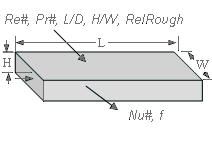Contents - Index

DuctFlow_NDProcedure DuctFlow_ND(Re, Pr, LoverD_h, Aspect, relRough: Nusselt_T,Nusselt_H, f) returns the average Nusselt number assuming constant temperature wall and constant heat flux for a given flow condition in a rectangular duct characterized by Reynolds and Prandtl numbers, length/hydraulic diameter, aspect ratio, and relative roughness.  The procedure also returns the friction factor for these conditions.   The procedure can be used for simultaneous thermal and hydrodynamic developing flow or fully-developed flow in the laminar, transitional or turbulent flow regimes.

Inputs:

Re - Reynolds number based on properties evaluated at the bulk average temperature

Pr - Prandtl number of the fluid evaluated at the bulk average temperature

LoverD_h - ratio of duct length to hydraulic diameter.

Aspect - ratio of the minimum to maximum dimension of duct.  This value should be <=1

RelRough - the ratio of the dispersions on the wall of the duct to the tube diameter (must be between 0 and 0.05)

Outputs:

Nusselt_T - Nusselt number assuming a constant wall temperature

Nusselt_H - Nusselt number assuming a constant wall heat flux

f -  apparent friction factor [-]

Notes:

This procedure  is called by DuctFlow.   It calls DuctFlow_Laminar and PipeFlow_Turbulent to obtain the appropriate Nusselt numbers and the friction factor.

The procedure will determine if the flow is laminar or turbulent.  Transitional flow is assumed to occur for Reynolds numbers (based on hydraulic diameter) between 2300 and 3000 and interpolation is applied between the laminar and turbulent correlations.

The parameter  LoverD_h is used to apply a developing flow correction if necessary.  Set LoverD_h to a large number if developing flow corrections are not applicable.  Values of L0verD_h < 0.1 are set to 0.1.

Two Nusselt numbers are returned.  Nusselt_T corresponds to a constant temperature wall and Nusselt_H corresponds to a constant heat flux.  These two values will be the same for turbulent flow.  For laminar flow, they provide upper and lower bounds on the Nusselt number as discussed in Section 5.2.4 of Nellis and Klein.

The procedure assumes simultaneous development of the thermal and hydrodynamic boundary layers; however, the results for a thermally developing/hydrodynamically developed laminar flow can be obtained by calling the procedure with a large Prandtl number.

Example:

Re=4500

Pr=2

Aspect=0.25

LoverD_h=20

relRough=0

Call ductflow_nd(Re,Pr,LoverD_h,Aspect,RelRough: Nusselt_T, Nusselt_H, f)

{Solution:

f=0.0433 [-]

Nusselt_H=24.96

Nusselt_T=24.96 [-]}